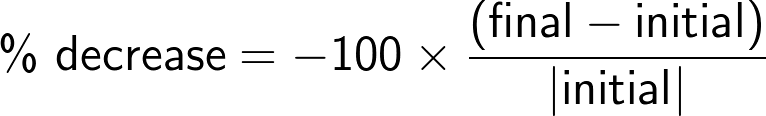# Percentage Decrease Calculator

By Mateusz Mucha and Dominik Czernia, PhD candidate
Last updated: Sep 11, 2021

The percentage decrease calculator determines the change from one amount to a lesser amount in terms of percent decrease. It is important to understand how to calculate percent decrease manually using the percent decrease formula. The content below will explain these concepts in further detail.

So you must provide an original quantity and a new quantity. The new quantity must be less than the original or the change will be an increase. The difference between the quantities is computed, which is divided by the original quantity. The result is multiplied by 100. This can also be accomplished using the percentage difference calculator and the percentage change calculator.

## How to calculate percent decrease?

To calculate percentage decrease between the original value `a` and new value `b`, follow these steps:

1. Find the difference between the original and new value: `a - b`.
2. Divide this difference by the original value: `(a - b) / a`.
3. Multiply the result by 100 to convert it into percentages.
4. That's it! As you see, it's not hard at all to calculate percent decrease.

## Percent decrease formula

The instruction we gave above can be summarized in the following math formula for percentage difference:

`Percent decrease = [(original_value - new_value)/original_value * 100]`

## What is an example of percentage decrease?

Suppose the original value is `750` and the new value is `590`. To compute the percentage decrease, perform the following steps:

1. Compute their difference `750 - 590 = 160`.
2. Divide `160` by `750` to get `0.213`.
3. Multiply `0.213` by `100` to get `21.3` percent.

The basic a concept of percentage is essentially the portion of one value in terms of another if the original was divided into 100 parts. For example, 3 compared to 50 is 6 compared to 100, which is 6 parts of 100, or 6 percent. The easy way to learn how to find a percentage is to take the partial quantity, divide it by the whole quantity, then multiply the remaining value by 100.

If you want to know how to calculate percent increase you can use the percent increase calculator or work it out by hand. Another interesting aspect of the percentage decrease calculator is that it displays the difference as well as the percent difference. In some cases, you may only want to know the raw difference, so we have designed the calculator to serve multiple purposes.

## FAQ

### What is the percentage decrease from 100 to 10?

The percentage decrease from 100 to 10 is equal to 90%. Indeed, we get: `(100 - 10) / 100 = 0.9` and `0.9 * 100% = 90%`, as we claimed.

### What is the percentage decrease from 5000 to 1000?

The percentage decrease from 5000 to 1000 is equal to 80%. Indeed, we get: `(5000 - 1000) / 5000 = 0.8` and `0.8 * 100% = 80%`, as we claimed.

Mateusz Mucha and Dominik Czernia, PhD candidateInitial value
Final value
Decrease
%
Difference
People also viewed…

Addiction calculator tells you how much shorter your life would be if you were addicted to alcohol, cigarettes, cocaine, methamphetamine, methadone, or heroin.

### Black Friday

How to get best deals on Black Friday? The struggle is real, let us help you with this Black Friday calculator!

### Parabola

The parabola calculator finds the vertex form, focus and directrix of every parabola.